# 2nd PUC Basic Maths Question Bank Chapter 1 Matrices and Determinants Ex 1.8

Students can Download Basic Maths Exercise 1.8 Questions and Answers, Notes Pdf, 2nd PUC Basic Maths Question Bank with Answers helps you to revise the complete Karnataka State Board Syllabus and score more marks in your examinations.

## Karnataka 2nd PUC Basic Maths Question Bank Chapter 1 Matrices and Determinants Ex 1.8

Part – A

2nd PUC Basic Maths Matrices and Determinants Ex 1.8 Two or Three Marks Questions and Answers

Question 1.
A man buys 5 apple, 6 mangoes and 7 oranges The cost of each apple is ₹ 4, each mango is ₹ 3 and each orange is ₹ 2. Represent the above in the matrix form and find tile total cost.
Let Q = Sale matrix = [5 6 7]
p = Price Matrix = $$\left[ \begin{matrix} 4 \\ 3 \\ 2 \end{matrix} \right]$$
Q.P = $$\left[ \begin{matrix} 5 & 6 & 7 \end{matrix} \right] \left[ \begin{matrix} 4 \\ 3 \\ 2 \end{matrix} \right]$$ = [20+18+14] = 
∴ Total cost = ₹ 52.

Question 2.
Bhavana buys 8 dozen of mangoes. 10 dozen of apples and 4 dozen of bananas. Mangoes cost ₹ 18 per dozen, apples cost ₹9 per dozen and bananas cost ₹6 per dozen. Find the total cost by matrix method.
Let Q = [8 10 4] p = $$\left[ \begin{matrix} 18 \\ 9 \\ 6 \end{matrix} \right]$$
∴ Total cost = Q.P = [8 10 4] P = $$\left[ \begin{matrix} 18 \\ 9 \\ 6 \end{matrix} \right]$$
= [144 + 90 + 24] = 
∴ Total cost = ₹258Question 3.
A manufacturer Renuka produces 3 products A, B and C which are sold in Delhi and Kolkata. The annual sales of these products are given below: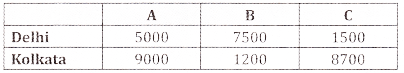It the sale price of the products A, B and C per unit be ₹2, ₹3 and ₹4 respectively. Calculate the total revenue in each centre by using matrix.∴ Total revenue for Delhi = ₹ 38500
Total revenue for Kolkotta = ₹ 56400.

Part – B

2nd PUC Basic Maths Matrices and Determinants Ex 1.8 Six Marks Questions and Answers

Question 1.
The monthly expenditure in an office for 3 months is given in the table.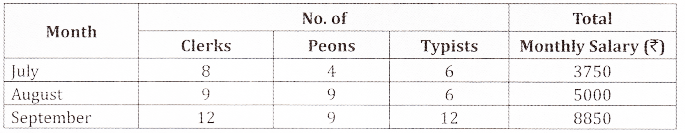Assuming that the salary br the Ultierent categories ob the stall did not vary from month to month. Calculate the salary for each type of staff per month using matrix method.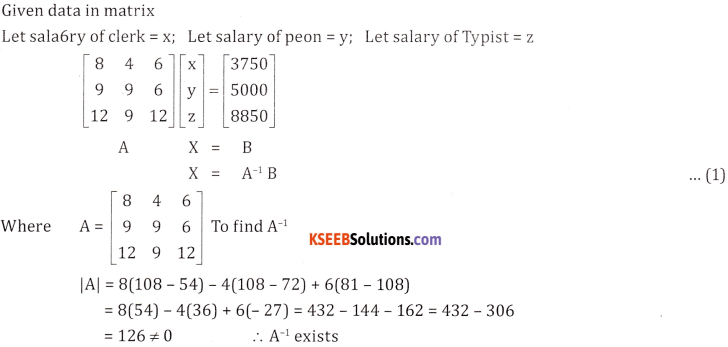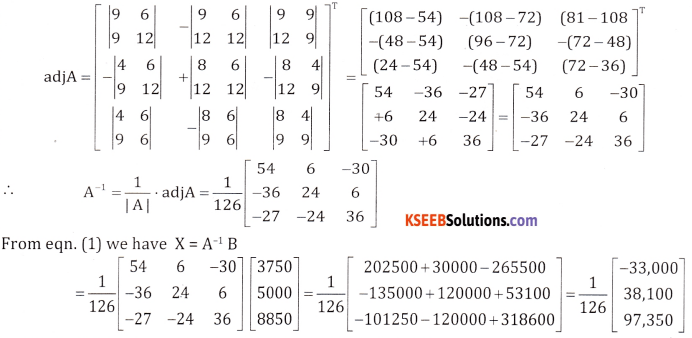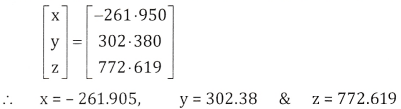Question 2.
Two farmers Preethi and Nithya cultivate only three varieties of rice namely. Basmati, permal & Naura. The sale (in rupees) of these varieties of rice by both farmers in the month of September and October are given by the following matrices A and B. September sale (in rupees).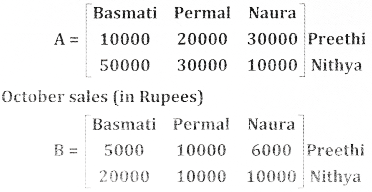(a) Find the combined sales in September and October for each tarmer in each variety
(b) Find the decrease in sales from September to October.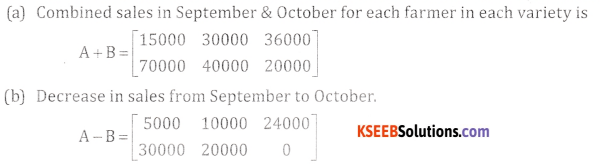Question 3.
In a legIslative assembly election, a political party hired a public relations firm to promote its candidate in three ways: telephone, house calls and letters. The cost per contact (in paise) is given in matrix A as: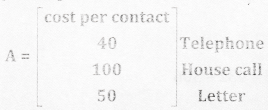The number of contacts of each type made in two cities X and Y is given byFind the tatal amount spent by the group in the two cities X and Y.
Total amount spent by the group in the two cities x & y = BA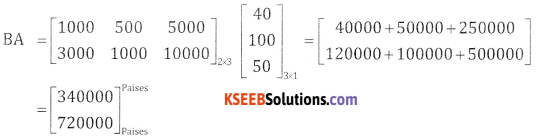Total amount in city X = ₹3400
Total amount in city Y = ₹7200Question 4.
The bookshop of a particular school has 10 dozen chemistry books, 8 dozen physics books, 10 dozen economics books. Their selling prices are ‘80, ‘60 and ‘40 each respectively. Find the total amount thebookshop will receive from selling all the books using matrix algebra.
Let Q = [10 8 10] & P = $$\left[ \begin{matrix} 80 \\ 60 \\ 40 \end{matrix} \right]$$
Total amount = Q.P = $$\left[ \begin{matrix} 10 & 8 & 10 \end{matrix} \right] \left[ \begin{matrix} 80 \\ 60 \\ 40 \end{matrix} \right]$$